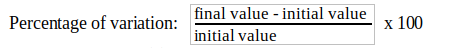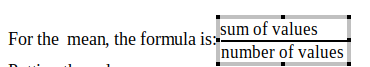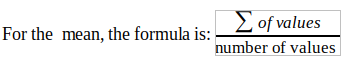How can we center text in Fraction inside Formulas object?

Hello!

I’ve inserted this formula in Libreoffice Writer:By writing in the formula editor:
{"final value - initial value"} over {"initial value"}

I’d like the bottom part of the fraction (“initial value”) to be centered.
How can I do that?
Thank you!

By the way, I’ve noticed that contrary to text, numbers in fraction are automatically centered.

then treat it as a value, without quotes around:
{final value " - " initial value} over {initial value}
or maybe:
{final value - initial value} over {initial value}
within *100:
{final value " - " initial value} over {initial value}" "*" "100

2 Likes

Ok, thank you. It’s working for this very specific case. But I have other formula where, for example, the numerator needs really to be considered as a text, not as a value:

For example, this formula:
{"Sum of values"} over {"number of values"}If I remove the quotes
{Sum of values} over {"number of values"}

This is what I get:which is not what I intended.

{alignc "Sum of values"} over {alignc "number of values"}

Thanks, that was it!
For other people searching it, the doc for this is here: Format - LibreOffice Help